# Texas Go Math Grade 5 Lesson 13.6 Answer Key Metric Measures

Refer to our Texas Go Math Grade 5 Answer Key Pdf to score good marks in the exams. Test yourself by practicing the problems from Texas Go Math Grade 5 Lesson 13.6 Answer Key Metric Measures.

## Texas Go Math Grade 5 Lesson 13.6 Answer Key Metric Measures

Essential Question
How can you compare and convert metric units?
To convert from one unit to another within the metric system usually means moving a decimal point. If you can remember what the prefixes mean, you can convert within the metric system relatively easily by simply multiplying or dividing the number by the value of the prefix.

Unlock the Problem

Using a map, Alex estimates the distance between his house and his grandparent’s house to be about 15,000 meters. About how many kilometers away from his grandparent’s house does Alex live?
The metric system is based on place value. Length, capacity, and mass each have a base unit (meter, liter, or gram) and use prefixes to show the relationship between larger and smaller units.

One Way Conversions Convert 15,000 meters to kilometers.Step 1
Find the relationship between the units.
There are 1,000 meters in 1 kilometer.
Step 2
Determine the operation to be used.
I am converting from a meter unit to a Kilometer unit, so I will divide.
Step 3
ConvertSo,
Alex’s house is 15 kilometers from his grand parent’s house.

Math Talk
Mathematical Processes
Choose two units in the chart. Explain how the two units are related.
The given chart is:Now,
From the given chart,
We can observe that
The two units are: Kilogram and Gram
Now,
We know that,
The relationship between 2 units are:
1 Kilogram = 1,000 Grams
Hence, from the above,
We can conclude that
The relationship between 2 units are:
1 Kilogram = 1,000 GramsAnother Way
Use a diagram.
Jamie made a bracelet 1.8 decimeters long. How many millimeters long is Jamie’s bracelet?

Convert 1.8 decimeters to millimeters.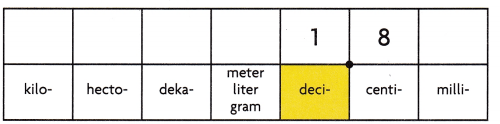Step 1
Show 1.8 decimeters.
Since the unit is decimeters, place the decimal point so that decimeters are the whole number unit.Step 2
Convert.
Cross out the decimal point and rewrite it so that millimeters will be the whole number unit. Write a zero to the left of the decimal point to complete the whole number.
So,
1 decimeter = 100 millimeters

Step 3
Record the value with the new unit.
1.8 dm = 180 mm
So,
Jamie’s bracelet is 180 millimeters long.

Try This! Complete the equation to show the conversion.

A.
Convert 247 milligrams to centigrams, decigrams, and grams.
Are the units being converted to a larger unit or a smaller unit?
The units are being converted to a smaller unit

Should you multiply or divide to convert?
You should divide to convert
Now,
247 mg ÷ 10 = 24.7 cg
247 mg ÷ 100 = 2.47 dg
247 mg ÷ 1,000 = 0.247 g

B. Convert 3.9 hectoliters to dekaliters, liters, and deciliters.
Are the units being converted to a larger unit or a smaller unit?
The units are being converted to a larger unit

Should you multiply or divide to convert?
You should multiply to convert
Now,
3.9 hL × 10 = 39 daL
3.9hL × 100 = 390 L
3.9 hL × 1,000 = _3,900 dL

Share and Show

Complete the equation to show the conversion.

Question 1.
8.47 L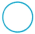10 = ____ dL
8.47 L10 = ____ cL
8.47 L1,000 = ____ mL
We know that,
1 Liter = 10 Deciliters
1 Liter = 100 Centiliters
1 Liter = 1,000 Milliliters
Hence, from the above,
We can conclude that
8.47 L × 10 = 84.7 dL
8.47 L × 100 = 847 cL
8.47 L × 1,000 = 8,470 mL

Think: Are the units being converted to a larger unit or a smaller unit?
If the units are converted from a larger unit to a smaller unit, divide
If the units are converted from a smaller unit to a larger unit, multiply

9,824 dg10 = ___ g
9,824 dg100 = ___ dag
9,824 dg1,000 = __ hg
We know that,
1 dg = 0.1 g
1 dg = 0.01 dag
1 dg = 0.001 hg
Hence, from the above,
We can conclude that
9,824 dg ÷ 10 = 982.4 g
9,824 dg ÷ 100 = 98.24 dag
9,824 dg ÷ 1,000 = _9.824 hg

Convert.
Question 3.
4,250 cm = ____ m
We know that,
1 cm = 0.01 m
So,
4,250 cm = 4,250 × 0.01
= 42.5 m
Hence, from the above,
We can conclude that
4,250 cm = 42.5 m

Question 4.
6,000 mL = ____ L
We know that,
1 mL = 0.001 L
So,
6,000 mL = 6,000 × 0.001 L
= 6 L
Hence, from the above,
We can conclude that
6,000 mL = 6 L

Question 5.
4 dg = ____ cg
We know that,
1 dg = 10 CG
So,
4 dg = 4 × 10 CG
= 40 CG
Hence, from the above,
We can conclude that
4 dg = 40 CG

Problem Solving

Practices Copy and Solve Compare. Write <, >, or =.

Question 6.
32 hg3.2 kg
We know that,
1 kg = 10 hg
So,
3.2 kg = 3.2 × 10 hg
= 32 hg
So,
32 hg = 32 hg
Hence, from the above,
We can conclude that6 km660 m
We know that,
1 km = 1,000 m
So,
6 km = 6 × 1,000 m
= 6,000 m
So,
6,000 m > 660 m
Hence, from the above,
We can conclude thatQuestion 8.
525 mL525 cL
We know that,
1 mL = 0.1 cL
So,
525 mL = 525 × 0.1 cL
= 52.5 cL
So,
52.5 cL < 525 cL
Hence, from the above,
We can conclude that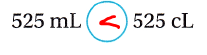Question 9.
Analyze How can you compare 4.25 decimeters and 4.25 centimeters without converting?
We know that,
1 dm = 10 cm
So,
4.25 decimeters = 4.25 × 10 centimeters
= 42.5 centimeters
So,
42.5 cm > 4.25 cm
Hence, from the above,
We can conclude that
4.25 decimeters are greater than 4.25 centimetersProblem Solving

For 10-11, use the table.

Question 10.
Multi-Step Kelly made one batch of peanut and pretzel snack mix. How many grams does she need to add to the snack mix to make 2 kilograms?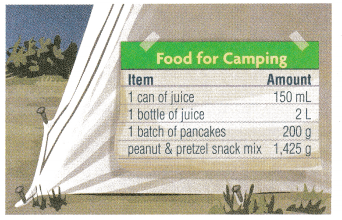It is given that
Kelly made one batch of peanut and pretzel snack mix
Now,
The given table is:Now,
From the above table,
We can observe that
The weight of one batch of peanut and pretzel snack mix is: 1,425 g
Now,
We know that,
1 kg = 1,000 g
So,
2 kg = 2,000 g
Now,
According to the given information,
The number of grams does Kelly needed to add to the snack mix to make 2 kilograms = 2,000 – 1,425
= 575 grams
Hence, from the above,
We can conclude that
The number of grams does Kelly needed to add to the snack mix to make 2 kilograms is: 575 grams

H.O.T. Multi-Step Kelly plans to take juice on her camping trip. Which will hold more juice, 8 cans or 2 bottles? How much more?It is given that
Kelly plans to take the juice on her camping trip
Now,
The given table is:Now,
From the above,
We can observe that
The capacity of 1 can of juice is: 150 mL
The capacity of 1 bottle of juice is: 2 L
Now,
We know that,
1 L = 1,000 mL
So,
2 L = 2 × 1,000 mL
= 2,000 mL
So,
150 mL < 2,000 mL
Now,
The difference between the capacities of a can of juice and a bottle of the juice = 2,000 – 150
= 1,850 mL
Hence, from the above,
We can conclude that
1 bottle of juice will hold more juice
The bottle of juice holds 1,850 mL more than the can of the juice

Question 12.
Apply A snowboard instructor has 4.2 liters of liquid wax for waxing snowboards. If she uses 1 milliliter for each snowboard, how many snowboards can she wax?
(A) 4
(B) 42
(C) 420
(D) 4,200
It is given that
A snowboard instructor has 4.2 liters of liquid wax for waxing snowboards
Now,
We know that,
1 Liter = 1,000 mL
So,
The given amount of liquid wax for waxing snowboards = 4.2 ×1,000 mL
= 4,200 mL
So,
The number of snowboards can the snowboard instructor can wax = $$\frac{4,200}{1}$$
= 4,2000 snowboards
Hence, from the above,
We can conclude that
The number of snowboards can the snowboard instructor can wax is:Daija wants to trim 3.5 centimeters from her hair. How should she move the decimal point to convert this number to millimeters?
(A) 1 place to the left
(B) 1 place to the right
(C) 2 places to the left
(D) 2 places to the right
It is given that
Daija wants to trim 3.5 centimeters from her hair
Now,
We know that,
1 centimeter = 10 millimeter
So,
3.5 centimeters = 3.5 × 10
= 35 mm
Hence, from the above,
We can conclude that
The way Daija moves the decimal point to convert this number to millimeters is:Question 14.
Multi-Step Vincent mixed 500 grams of raisins, 400 grams of dried cranberries, and 300 grams of almonds to make Trail Mix. How many grams of cashews must he add to make 2 kilograms of Trail Mix?
(A) 140 grams
(B) 200 grams
(C) 800 grams
(D) 1,200 grams
It is given that
Vincent mixed 500 grams of raisins, 400 grams of dried cranberries, and 300 grams of almonds to Make Trail Mix
So,
The total amount of Trail Mix = 500 + 400 + 300
= 1,200 grams
Now,
We know that,
1 kilogram = 1,000 grams
So,
2 kilograms = 2,000 grams
Now,
The number of grams of cashews must be added to make 2 kilograms of Trail Mix = 2,000 – 1,200
= 800 grams
Hence, from the above,
We can conclude that
The number of grams of cashews that must be added to make 2 kilograms of Trail Mix is: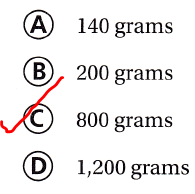Texas Test Prep

Monica has 426 millimeters of fabric. How many centimeters of fabric does Monica have?
(A) 4,260 centimeters
(B) 42.6 centimeters
(C) 4.26 centimeters
(D) 0.426 centimeters
It is given that
Monica has 426 millimeters of fabric
Now,
We know that,
1 millimeter = 0.1 centimeter
So,
426 millimeters = 426 × 0.1 centimeter
= 42.6 centimeters
Hence, from the above,
We can conclude that
The number of centimeters of fabric does Monica have is:### Texas Go Math Grade 5 Lesson 13.6 Homework and Practice Answer Key

Convert.

Question 1.
350 cm = ___ m
We know that,
1 cm = 0.01 m
So,
350 cm = 350× 0.01
= 3.5 m
Hence, from the above,
We can conclude that
350 cm = 3.5 m

Question 2.
4,000 mL = __ L
We know that,
1 mL = 0.001 L
So,
4,000 mL = 4,000 × 0.001
= 4 L
Hence, from the above,
We can conclude that
4,000 mL = 4 L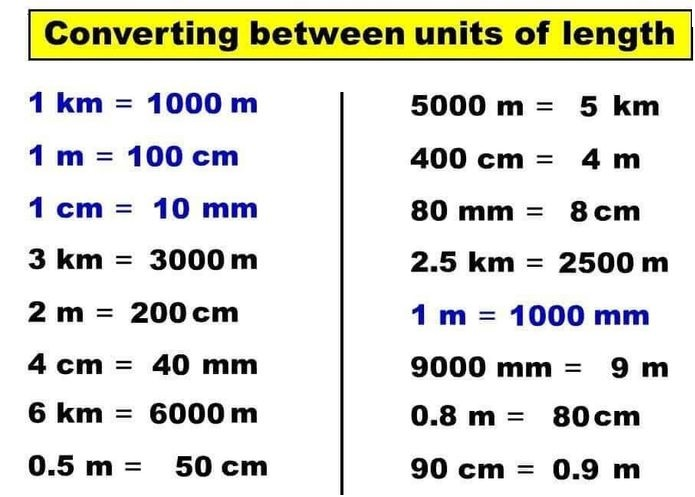Question 3.
62 dg = ____ cg
We know that,
1 dg = 10 cg
So,
62 dg = 62 × 10
= 620 cg
Hence, from the above,
We can conclude that
62 dg = 620 cg

9 m = ___ mm
We know that,
1 m = 1,000 mm
So,
9 m = 9 × 1,000
= 9,000 mm
Hence, from the above,
We can conclude that
9 m = 9,000 mm

Question 5.
150 g = ___ hg
We know that,
1 g = 0.01 hg
So,
150 g = 150 × 0.01 hg
= 1.5 hg
Hence, from the above,
We can conclude that
150 g = 1.5 hg

Question 6.
16 L = __ hL
We know that,
1 L = 0.01 hL
So,
16 L = 16 × 0.01 hL
= 0.16 hL
Hence, from the above,
We can conclude that
16 L = 0.16 hL

Compare. Write <, >, or .

Question 7.
6 km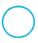54,000 mm
We know that,
1 km = 10,00,000 mm
So,
6 km= 6 × 10,00,000 mm
= 60,00,000 mm
So,
60,00,000 mm > 54,000 mm
Hence, from the above,
We can conclude that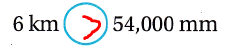Question 8.
3 KL30,000 L
We know that,
1 kL = 1,000 L
So,
3 kL= 3 × 1,000 L
= 3,000 L
So,
3,000 L < 30,000 L
Hence, from the above,
We can conclude that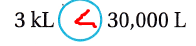Question 9.
20g0.02 kg
We know that,
1 g = 0.001 Kg
So,
20 g= 20 × 0.001 Kg
= 0.02 kg
So,
0.02 g = 0.02 g
Hence, from the above,
We can conclude that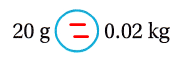52L5,200 mL
We know that,
1 L = 1,000 mL
So,
52 L= 52 × 1,000 mL
= 52,000 mL
So,
52,000 mL > 5,200 mL
Hence, from the above,
We can conclude thatQuestion 11.
14m140 cm
We know that,
1 m = 100 cm
So,
14 m= 14 × 100 cm
= 1,400 cm
So,
1,400 cm > 140 cm
Hence, from the above,
We can conclude that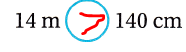Question 12.
312 mg312 dg
We know that,
1 mg = 0.01 dg
So,
312 mg= 312 × 0.01 dg
= 3.12 dg
So,
3.12 dg < 312 dg
Hence, from the above,
We can conclude that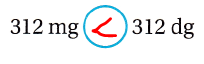Question 13.
Is 25 milligrams lighter or heavier than 25 centigrams? Explain how you know.
We know that,
1 mg = 0.1 cg
So,
25 mg = 25 × 0.1 cg
= 2.5 cg
So,
2.5 cg < 25 cg
Hence, from the above,
We can conclude that
25 milligrams is lighter than 25 centigrams

Problem Solving

Question 14.
Lucy and Donavan measured the length of the school garden. Lucy’s measurement is 11.3 meters and Donavan’s measurement is 113 centimeters. Could both be correct? Explain.
It is given that
Lucy and Donavan measured the length of the school garden. Lucy’s measurement is 11.3 meters and Donavan’s measurement is 113 centimeters
Now,
We know that,
1 meter = 100 centimeters
So,
11.3 meters = 11.3 × 100 centimeters
= 1,130 centimeters
So,
1,130 centimeters > 113 centimeters
Hence, from the above,
We can conclude that
Lucy and Donavan are not correct
Lucy’s measurement is greater than Donavan’s measurement

Question 15.
Shanika makes 2,800 milliliters of chicken soup for a dinner party. She needs 3.5 liters. How many more milliliters does Shanika need to make? How many liters is that?
It is given that
Shanika makes 2,800 milliliters of chicken soup for a dinner party. She needs 3.5 liters
Now,
We know that,
1 L = 1,000 mL
So,
2,800 mL = 2,800 × $$\frac{1}{1,000}$$
= 280 L
3.5 L = 3.5 × 1,000
= 3,500 mL
So,
The number of more milliliters does Shanika need to make = 3,500 – 2,800
= 700 mL
= 700 × $$\frac{1}{1,000}$$ L
= 70 L
Hence, from the above,
We can conclude that
The number of more milliliters does Shanika need to make is: 700 mL (or) 70 L

Texas Test Prep

Lesson Check

Question 16.
Jordan has 3.2 liters of paint left after painting the scenery for the school play. How can she convert this number to find how many milliliters of paint she has left?
(A) Multiply by 100.
(B) Multiply by 1,000.
(C) Divide by 100.
(D) Divide by 1,000.
It is given that
Jordan has 3.2 liters of paint left after painting the scenery for the school play
Now,
We know that,
1 L = 1,000 mL
So,
3.2 L = 3.2 × 1,000 mL
Hence, from the above,
We can conclude that
Jordan can convert this number to find the number of milliliters of paint she has left by:5th Grade Metric Conversion Chart Go Math Lesson 13.6 Answer Key Question 17.
Martin needs to ship a box that weighs 12,000 grams. How should he move the decimal point to convert this number to kilograms?
(A) 2 places to the right
(B) 3 places to the right
(C) 2 places to the left
(D) 3 places to the left
It is given that
Martin needs to ship a box that weighs 12,000 grams
Now,
We know that,
1 kg = 1,000 grams
So,
12,000 grams = 12,000 × $$\frac{1}{1,000}$$
= 12 kg
Hence, from the above,
We can conclude that
Martin has to move the decimal point to convert this number to kilograms by:Question 18.
Akeem has 24 meters of rope. Which of the following lengths is equal to 24 meters?
(A) 0.024 kilometer
(B) 0.24 kilometer
(C) 2.4 kilometers
(D) 240 kilometers
It is given that
Akeem has 24 meters of rope
Now,
We know that,
1 meter = 0.001 kilometers
So,
24 meters = 24 × 0.001 kilometers
= 0.024 kilometers
Hence, from the above,
We can conclude that
The following lengths that is equal to 24 meters is: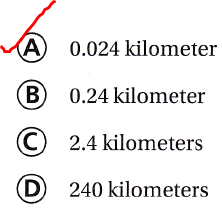Question 19.
A pastry chef bought a 0.26-liter container of vanilla extract for baking. If she uses 1 milliliter of vanilla in each batch of pastries, how many batches can she make?
(A) 2,600
(B) 260
(C) 26
(D) 2.6
It is given that
A pastry chef bought a 0.26-liter container of vanilla extract for baking and she uses 1 milliliter of vanilla in each batch of pastries
Now,
We know that,
1 Liter = 1,000 mL
So,
0.26 Liters = 0.26 × 1,000
= 260 mL
So,
The number of batches of vanilla that pastry chef can make = $$\frac{260}{1}$$
= 260 batches
Hence, from the above,
We can conclude that
The number of batches of vanilla that pastry chef can make is: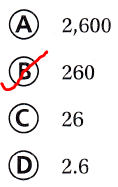Question 20.
Multi-Step The weight limit for luggage at the airport is 22 kilograms per person. Rico packs two suitcases and finds their weights to be 8,500 grams and 9,200 grams. How many more grams can he pack without going over the weight limit?
(A) 4,300 grams
(B) 5,700 grams
(C) 13,500 grams
(D) 17,700 grams
It is given that
The weight limit for luggage at the airport is 22 kilograms per person. Rico packs two suitcases and finds their weights to be 8,500 grams and 9,200 grams
Now,
We know that,
1 kilogram = 1,000 grams
So,
The weight limit for luggage at the airport per person in grams = 22 × 1,000
= 22,000 grams
So,
The number of more grams cRico can pack without going over the weight limit = 22,000 – (8,500 + 9,200)
= 22,000 – 17,700
= 4,300 grams
Hence, from the above,
We can conclude that
The number of more grams cRico can pack without going over the weight limit is: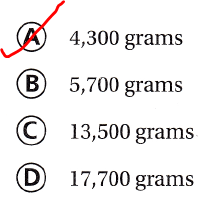Question 21.
Multi-Step The county repairs three sections of a road. The first section is 2.5 kilometers long, the second section is 12 hectometers long, and the third section is 800 meters long. What is the total length of the repaired sections of the road?
(A) 3.5 kilometers
(B) 4.0 kilometers
(C) 4.5 kilometers
(D) 0.5 kilometer
It is given that
The county repairs three sections of a road. The first section is 2.5 kilometers long, the second section is 12 hectometers long, and the third section is 800 meters long
Now,
We know that,
1 hm = 0.1 km
1m = 0.001 km
So,
The total length of the repaired sections of the road = 2.5 + (12 × 0.1) + (800 × 0.001)
= 2.5 + 1.2 + 0.8
= 4.5 kilometers
Hence, from the above,
We can conclude that
The total length of the repaired sections of the road is: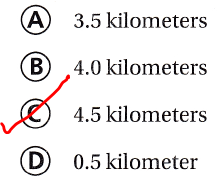Scroll to Top